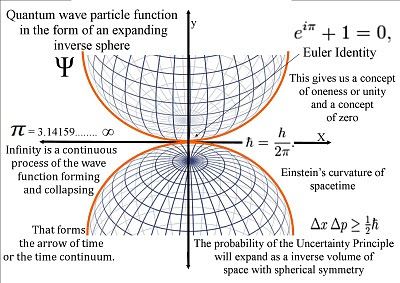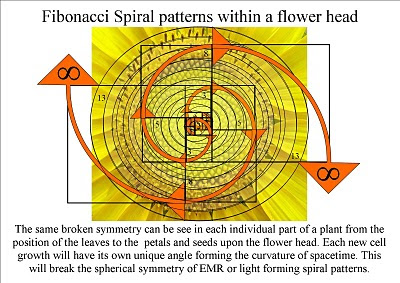Sunday, 19 September 2010

A new theory on how Schrödinger’s wave equation represents the forward passage of time itself photon by photon or moment by moment.

~
A link to the video 'A reason for mathematical infinity an artist theory on spacetime'

In this video mathematical infinity is explained as a physical process unfolding at the quantum level of the atoms. That continuously forms the arrow of time and geometry of spacetime.

This theory (Quantum Atom Theory) is based on two simple postulates the first is that Schrödinger's wave equation does not just predicts what the wave-function will be in the future, but that this process represents the forward passage of time itself photon by photon or moment by moment.The second postulate is that Heisenberg’s Uncertainty Principle is the same uncertainty that we have with any future event.

Only infinity or an infinite process could create the diversity we see within our Universe. It is because time forms a time line from the past to the future that we have line symmetry in physics and in the physical world.

Everything that is part of this infinite process will have line symmetry including matter and antimatter, positive and negative charge there is even symmetry between electricity and magnetism forming electromagnetic fields.There is even line symmetry in the physical shape of life itself. This is because we are not independent of this process but are active participants in the formation of our own spacetime geometry forming our own time line.Schrödinger's wave-function expands as an inverse sphere the reason it is inverse is because we are within this process and any object observed from within will look inverse.On the level of our everyday life the wave-function expands as electromagnetic waves (light) that is itself inverse in the form of frequency and wavelength.The quantum particle wave-function forms a universal process of spherical symmetry forming and breaking that formed a geometrical and therefore mathematical base for Charles Darwin's theory on the evolution of life.In this theory the reason life never produces straight line but endless variety of curves and spirals is because of the physical shape of the wave-function is always forming Einstein’s curvature of spacetime.

This might sound like a half-baked idea, but this is not because it is based on pseudo science, but because of the weirdness of quantum mechanics.

~

Sunday, 12 September 2010

A physical reason for mathematical infinity

A link to videos on Quantum Atom Theory and artist theory on the Arrow of Time.

~

We have mathematical infinity in many forms. We have the infinite series of whole numbers and the infinite series of irrational numbers like pi.

There is also infinity between each whole number that can always be divided into ever smaller parts

If the laws of mathematics are true and linked to the law of nature and physics then these mathematical infinities must represent something in the physical reality of our Universe.

In a new theory called Quantum Atom Theory these mathematical infinities are formed by a continuous process at the quantum level.

Light or electromagnetic waves are continuously collapsing into photons of quantized energy forming new waves of future probability. We feel this process as the forward passage of time that form the geometry or curvature of spacetime.

It is because we can choose where and when to come in contact with the wave-function collapsing the light waves of EMR into quantum particles (photons) that we can create our own spacetime geometry this is why we have free-will.

The reason why we can always divide infinity into sets of infinities is because of this continuous process of the wave-particle function collapsing into new quantum particles of space and time. Because of this we have a continuous expansion at the quantum level that forms the continuum of time and the geometry of spacetime that can always be divided into sets of infinities.

The explanation is very simple a mathematician will continuously form his own spacetime geometry just like any other object in our Universe. Therefore it is only natural that he will be able to divide that geometry into infinitely smaller parts. We are all active participants in the dynamics of our Universe.

It is because this process is continuous that our number system is infinite and we have the infinite series of whole numbers. We have irrational numbers like pi because of the physical shape of the wave-function (spherical or inverse sphere) that forms the arrow of time.

In this theory Schrödinger’s equation does not just predict what the wave-function will be in the future, but this process represents the forward passage of time itself photon by photon or moment by moment.

The probability of the wave-function only works forward in time. We can always calculate backwards and see the position and momentum of a quantum particle in the past.

This is precisely the same as our everyday experience of nature and time, moving from a known past into an uncertain future!

Therefore Heisenberg’s Uncertainty Principle and the probability of the wave-function are the same uncertainty that we have with any future event.

I have tried to make this theory as simple as possibly in the belief that everyone should be able to understand it; a correct theory should be based on simplicity, symmetry and beauty.

~